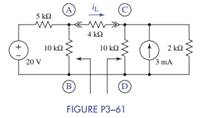### Create an Account

Home / Questions / The purpose of this problem is to use Thévenin equivalent circuits to find the current iL ...

# The purpose of this problem is to use Thévenin equivalent circuits to find the current iL in Figure P3−61 Find the Thévenin equivalent circuit seen looking to the left of terminals

The purpose of this problem is to use Thévenin equivalent circuits to find the current iL in Figure P3−61. Find the Thévenin equivalent circuit seen looking to the left of terminals A and B. Find the Thévenin equivalent circuit seen looking to the right of terminals C and D. Connect these equivalent circuits together with the load resistor and find the current iL.Jun 15 2020 View more View LessSubscribe To Get Solution